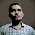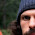## Tuesday, May 8, 2018

### Just published: "Rejecting or Accepting Parameter Values in Bayesian Estimation"

Just published: "Rejecting or Accepting Parameter Values in Bayesian Estimation" in the journal, Advances in Methods and Practices in Psychological Science.

Abstract: This article explains a decision rule that uses Bayesian posterior distributions as the basis for accepting or rejecting null values of parameters. This decision rule focuses on the range of plausible values indicated by the highest density interval of the posterior distribution and the relation between this range and a region of practical equivalence (ROPE) around the null value. The article also discusses considerations for setting the limits of a ROPE and emphasizes that analogous considerations apply to setting the decision thresholds for p values and Bayes factors.Figure 1 of the article.

From the introduction:
In everyday life and in science, people often gather data to estimate a value precisely enough to take action. We use sensory data to decide that a fruit is ripe enough to be tasty but not overripe—that the ripeness is “just right” (e.g., Kappel, Fisher-Fleming, & Hogue, 1995, 1996). Scientists measured the position of the planet Mercury (among other things) until the estimate of the parameter γ in competing theories of gravity was sufficiently close to 1.0 to accept general relativity for applied purposes (e.g., Will, 2014).

These examples illustrate a method for decision making that I formalize in this article. This method, which is based on Bayesian estimation of parameters, uses two key ingredients. The first ingredient is a summary of certainty about the measurement. Because data are noisy, a larger set of data provides greater certainty about the estimated value of measurement. Certainty is expressed by a confidence interval in frequentist statistics and by a highest density interval (HDI) in Bayesian statistics. The HDI summarizes the range of most credible values of a measurement. The second key ingredient in the decision method is a range of parameter values that is good enough for practical purposes. This range is called the region of practical equivalence (ROPE). The decision rule, which I refer to as the HDI+ROPE decision rule, is intuitively straightforward: If the entire HDI—that is, all the most credible values—falls within the ROPE, then accept the target value for practical purposes. If the entire HDI falls outside the ROPE, then reject the target value. Otherwise, withhold a decision.

In this article, I explain the HDI+ROPE decision rule and provide examples. I then discuss considerations for setting the limits of a ROPE and explain that similar considerations apply to setting the decision thresholds for p values and Bayes factors.
The published article is available here and a pre-print version, with some differences in details, is available here .

1.Hello, Mr. Kruschke, good night.

In your robust models, I see you use an individual mu and sigma for each distribution, but always a shared nu for both. Why not an individual nu for each distribution? Are there cases when one should use an individual nu for each distribution?

1.It would be straight forward to give each group its own nu, analogous to giving each group its own sigma. However, the value of nu is influenced mainly by the outlying data, and typically each group has relatively small numbers of outlying data. Therefore the estimate of nu is typically very uncertain for each group. By using one nu for all groups, we are using the outliers from all groups to inform the tail-heaviness of the noise distribution. Using one nu for all groups might be interpreted as assuming that the same outlier process affects all groups (whatever that means). If you have a situation in which you have a ton of data in the tails and reason to believe that the normalities of the groups differ, then do give each group its own nu parameter.

2.P.S. If you haven't seen it, be sure to read this blog post about the values of nu: https://doingbayesiandataanalysis.blogspot.com/2015/12/prior-on-df-normality-parameter-in-t.html

2.Really nice, simple to understand paper.

There's one thing that I'm not sure I can get my head around:
Is there a particular reason for using the location of the HDI rather than simply asking how much of the distribution falls above vs below the upper bound of the ROPE? Why ignore the density in the outer tails when making the decision?

1.In the Supplement, at https://osf.io/fchdr/ , there's a section headed, "Decision rule based on ROPE alone." That might address your question. If not, let me know.

2.That is the discussion I was looking for, thanks.

The HDI+ROPE approach is probably better protection against the kinds of erroneous interpretations you describe, if you don't bother to look at the posterior. But I don't think I'd ever consider interpreting either result (HDI+ROPE or ROPE-only) without actually looking at the posterior distribution.